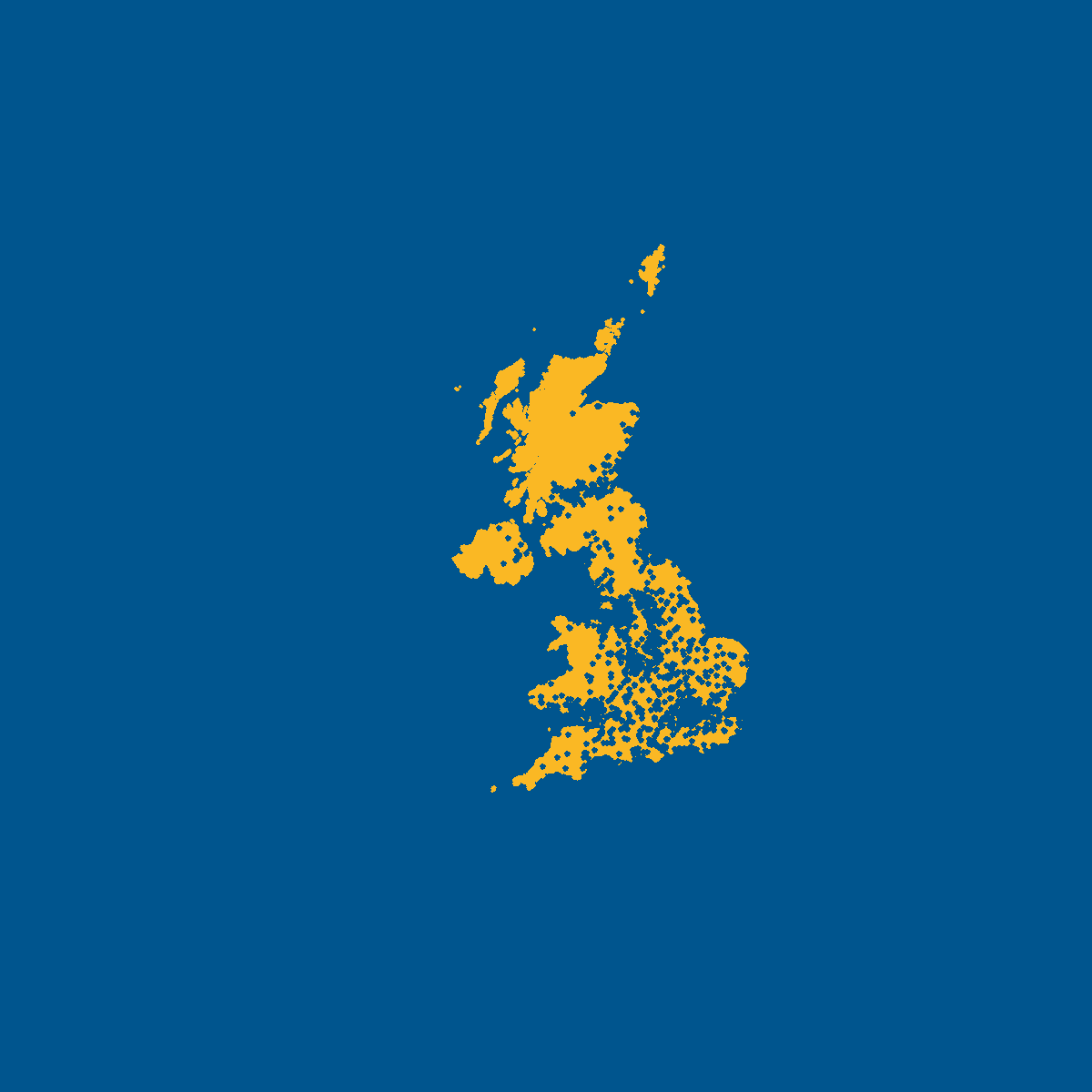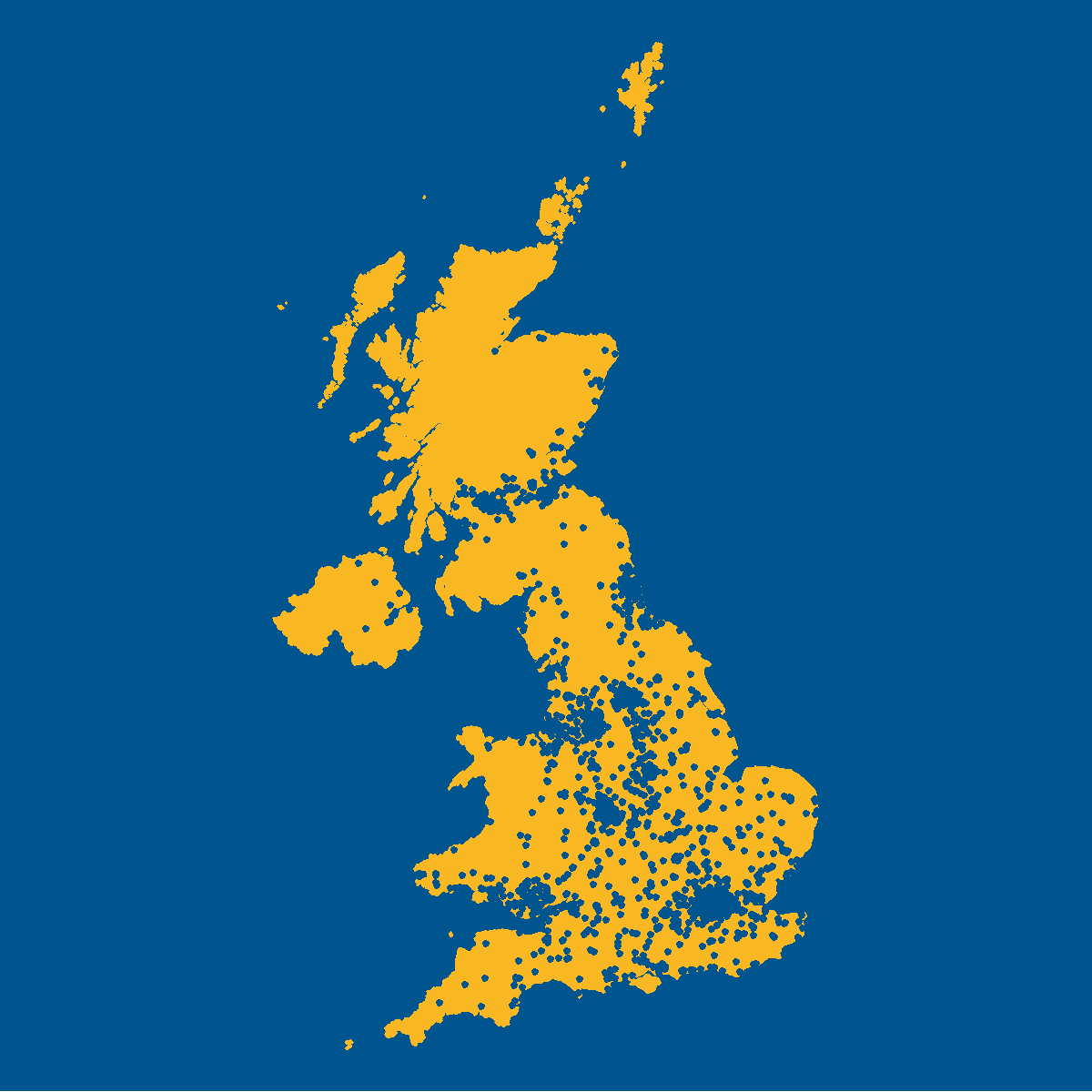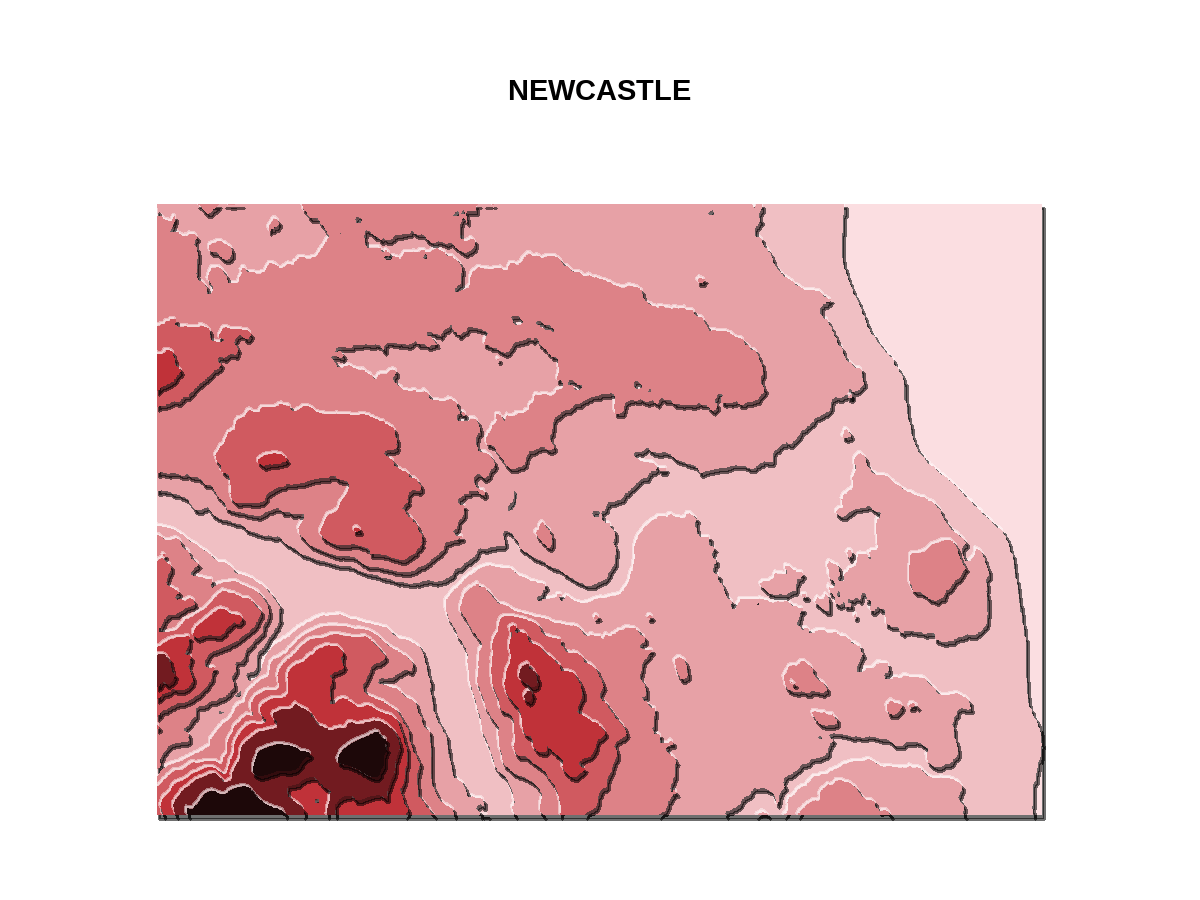Want to share your content on R-bloggers? click here if you have a blog, or here if you don't.

Throughout November I took part in the #30DayMapChallenge – a daily mapping, cartography, and data visualization challenge aimed at the spatial community. You can read my recap of the challenge in the blog I wrote last week, if you’re interested in finding out more about it. Throughout the challenge, I created most of my maps using R. This blog post details the R packages that I often find myself using the most often when I’m visualising spatial data – including some new ones I found out about during the #30DayMapChallenge!

I’ve split this blog post into two sections:

• Two dimensional maps: mapping latitude and longitude;
• Three dimensional maps: in addition to mapping latitude and longitude, you also want to map elevation.

## Two dimensional maps

To demonstrate how different packages work, I’m going to use two data sets that I used frequently throughout the #30DayMapChallenge:

You can read the data into R using the {sf} package (or another package of your choice!):

```uk <- sf::st_read("UK/CTRY_DEC_2021_UK_BUC.shp")
```

Initially the Greggs data set is a simple {tibble} rather than a spatial object, so you can convert it to an `sf` object. You also want to make sure that both data sets use the same coordinate reference system - here, I’ve changed the co-ordinate reference system of the Greggs data to use EPSG:27700 as the UK shapefiles already do.

```library(sf)
library(dplyr)
greggs_sf <- greggs %>%
st_as_sf(coords = c("lon", "lat")) %>%
st_set_crs(4326) %>%
st_transform(crs = 27700)
```

### Base R

So let’s make our first map! The simplest way to plot anything (including maps) in R is to use the base R `plot()` function.

```png("base.png", width = 4, height = 4, units = "in", res = 300)
par(bg = "#00558e")
plot(st_geometry(uk), col = "#fab824", border = "#fab824")
plot(st_geometry(greggs_sf), pch = 19, col = "#00558e", cex = 0.2, add = TRUE)
dev.off()
```

The base R map has a slightly odd default scaling in my opinion - to get the map to be focused on the UK a little more, and include less background space, I’d need to manually manipulate the axes limits. If we don’t use `st_geometry()` around our `sf` object, we’ll get multiple maps created - one for each other column in our data set.### {ggplot2}

I couldn’t talk about visualising data in R without mentioning {ggplot2}! If you’re working with `sf` objects, the `geom_sf()` function is your best friend - you don’t need to specific the latitude and longitude in the `aes()` function, it detects them automatically.

```library(ggplot2)
ggplot() +
geom_sf(data = uk,
linewidth = 0.5,
colour = "#fab824",
fill = "#fab824") +
geom_sf(data = greggs_sf,
size = 0.1,
colour = "#00558e") +
theme_void()
ggsave("ggplot2.png", height = 4, width = 4, bg = "#00558e")
```

If you wanted to, you could also specify co-ordinates in the `aes()` function and use `geom_point()` and `geom_polygon()` instead. Since {ggplot2} isn’t primarily designed for making maps, the default theme doesn’t usually look good - you can remove all theme elements with `theme_void()`.### {tmap}

The {tmap} package is designed for drawing thematic maps in R. {tmap} is similar to {ggplot2} in that the idea is to iteratively add layers to maps. In fact, the map I’ve recreated here looks identical to the one created above with {ggplot2}!

```library(tmap)
png("tmap.png", width = 4, height = 4, units = "in", res = 300)
tm_shape(uk_sf) +
tm_fill(col = "#fab824") +
tm_borders(col = "#fab824") +
tm_shape(greggs_sf) +
tm_dots(col = "#00558e") +
tm_layout(frame = FALSE, bg.color = "#00558e")
dev.off()
```

The difference is that {tmap} is created specifically for making maps. One of the functions in {tmap} that I really like is `tm_style()`: this allows you to add pre-defined styling to your maps, including colour schemes inspired by some common LaTeX themes that means you can match your maps to your presentations more easily!### {leaflet}

The {leaflet} R package provides an interface to Leaflet - an open-source JavaScript library for interactive maps. Here, I needed to change the co-ordinate reference system of my data before I plotted it, since {leaflet} expects data to use the World Geodetic System 1984 co-ordinate reference system.

```library(leaflet)
library(mapview)
new_uk <- uk_sf %>%  sf::st_transform(crs = 4326)
new_greggs <- greggs_sf %>%  sf::st_transform(crs = 4326)
m <- leaflet() %>%
stroke = FALSE,
fillOpacity = 1,
fillColor = "#fab824") %>%
fillOpacity = 1,
stroke = FALSE,
fillColor = "#00558e")
m
mapshot(m, file = "leaflet.png")
```

Although {leaflet} is primarily used to create interactive maps, the `mapshot()` function from {mapview} takes a static snapshot. I couldn’t find a built-in way to change the background colour of the map (which is light grey by default). Instead, I added a base map underneath with `addTiles()`. If you’re using a leaflet map with Quarto, R Markdown, or Shiny (which is likely since these maps are interactive), then you can edit the background colour using CSS styling.If you’re interested in adding background maps to static plots with {ggplot2}, I’d suggest looking at {ggmap} which provides background tiles from a variety of sources.

## Three dimensional maps

Alongside latitude and longitude it’s often of interest to visualise a third variable. This might be a true third dimension, i.e. elevation, or it could be a variable that you want to see the spatial changes in, e.g. rainfall. The examples here show elevation, but you could use the same tools to visualise more abstract third dimensions.

If you don’t have elevation data, you can use the {elevatr} R package to access elevation data from various sources. Here, I’ve provided a the co-ordinates of the minimum and maximum latitude and longitude of a bounding box around Newcastle, though you can also pass in a shapefile.

```library(elevatr)
elev_data <- get_elev_raster(
locations = data.frame(x = c(-1.760, -1.335), y = c(54.898, 55.067)),
z = 10,
prj = "EPSG:4326",
clip = "locations")
```

### Base R

Like many classes of objects, the `plot()` function understands how to plot raster objects.

```png("base_elevation.png", width = 4, height = 3, units = "in", res = 300)
par(mar = c(1, 1, 3, 1), bty = 'n')
plot(elev_data, axes = FALSE, horizontal = TRUE)
title(main = "NEWCASTLE",
cex.main = 1.8,
font.main = 2,
col.main = "black")
dev.off()
```

The base R plot here actually looks okay. The river is very clear, although in my opinion other colour palettes might be more appropriate - the yellow and the white both look lighter than the orange - but one represents higher elevation, the other lower.### {tanaka}

The {tanaka} R package implements a shaded contour lines method to improve the representation of topography on a map. Before using the `tanaka()` function, you need to convert the elevation data into a `SpatRaster` class of object, using the `rast()` function from {terra}.

```library(tanaka)
library(terra)
elev_raster <- rast(elev_data)
png("tanaka.png", width = 4, height = 3, units = "in", res = 300)
par(mar = c(1, 1, 3, 1))
tanaka(elev_raster, legend.pos = "n")
title(main = "NEWCASTLE",
cex.main = 1.8,
font.main = 2,
col.main = "black")
dev.off()
```

I really like {tanaka} contours - I find them much easier to interpret than flat contour lines, and the monochromatic colour palette is clearly shows the direction of change.If you’d rather have a create tanaka contours using {ggplot2}, then the {metR} package provides the `geom_contour_tanaka()` to do something similar in {ggplot2}.

The {rayshader} package is one of my favourite R packages! It’s designed to create 2D and 3D maps and plots. There are a lot of parameters to play with, and you can look at your spatial data from almost any angle!

```library(rayshader)
elev_mat <- raster_to_matrix(elev_data)
elev_mat %>%
plot_3d(elev_mat, zscale = 10, fov = 0, theta = 0, phi = 60,
windowsize = c(600, 450),
zoom = 0.7,
background = "lightgrey")
clear = FALSE,
title_text = "NEWCASTLE",
title_size = 50,
title_color = "white",
title_font = "serif")
```

The {rayshader} package has so many functions to add realistic elements to your map - including cloud cover using the `render_clouds()` function! If you’re interested in 3D visualisation in R, I’d also recommend checking out the {rayrender} package.### Final thoughts

There are lots of other R packages out there that are useful for visualising spatial data, and even more if you’re interested in manipulating and modelling spatial data - these are just of the R packages that I find myself using the most often. The Geocomputation with R book is an excellent reference for getting started in working with spatial data in R - and it’s freely available online!

You can also download a .R file with all the code used here from my website.